Don't Panic.Steve

# 神经网络的基础

## 神经网络表示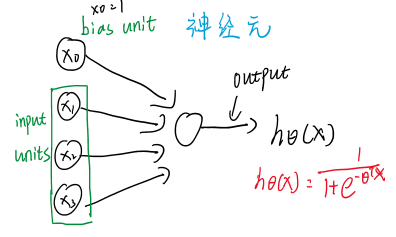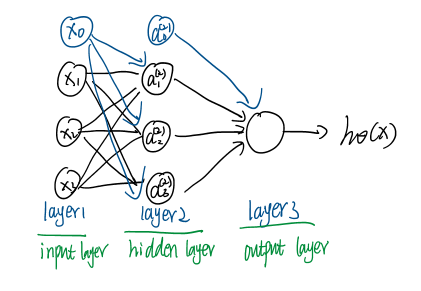• $a^{(j)}_i$:第 $j$ 层的第$i​$个激活单元
• $\theta^j_{ab}$ : 表示计算$j+1$层时第$a$项, 第$j$层第$b$项的参数
• $\theta^{(j)}$ : 将第$j$层变成第$j+1$层的参数
• $L$ : layer总数目 上图 $L = 3$
• $S_l$: 第l层由n个单位 如上图 $S_2=4$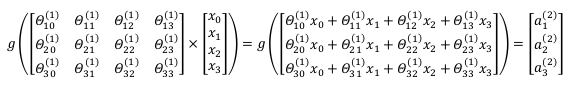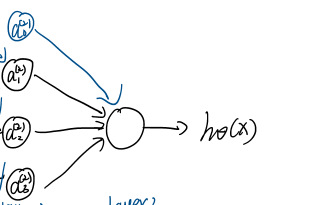## 偏置的作用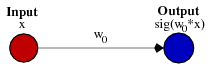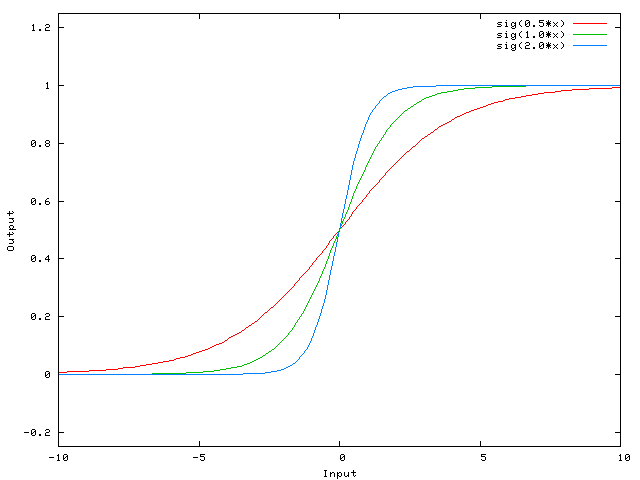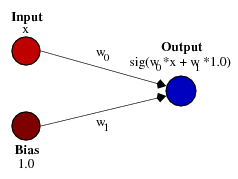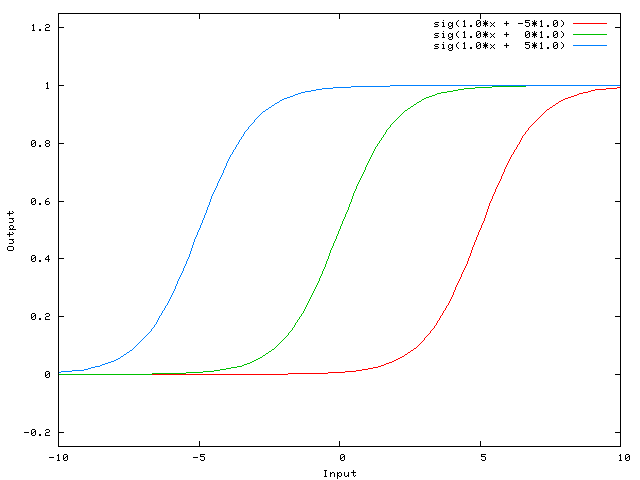## 多分类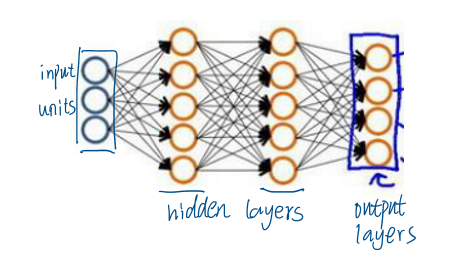# 神经网络的学习

## 反向传播算法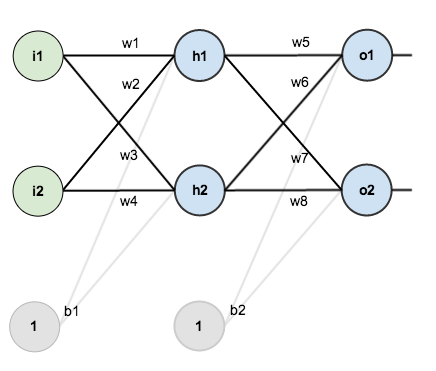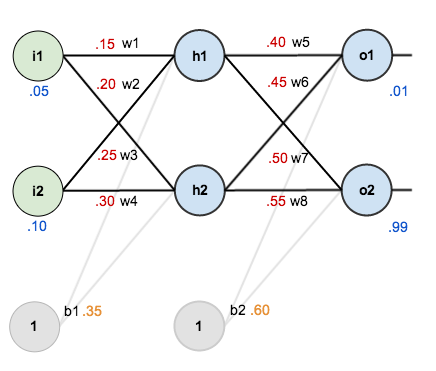### 1. 前向传播：

$o_1$是由两个项决定的：

### 3. 反向传播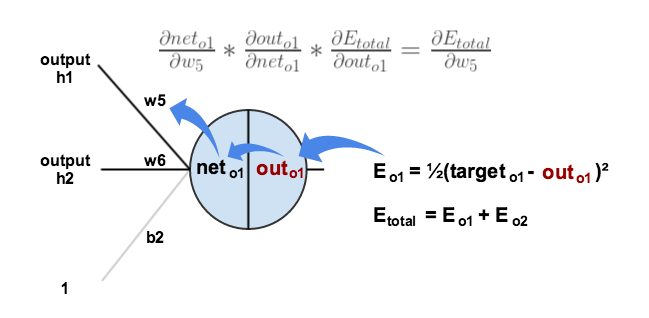$\frac { \partial E _ { \text { total } } } { \partial w _ { 5 } } = \delta _ { o 1 } out _ { h 1 }$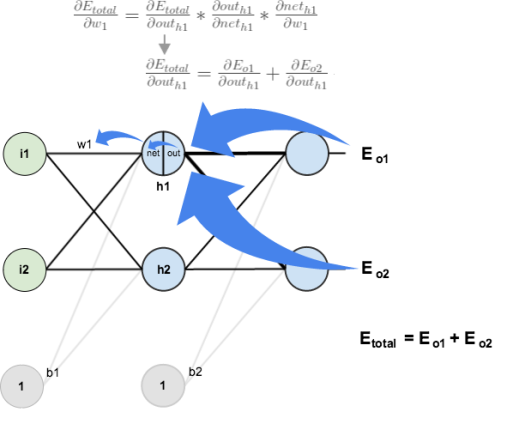$\frac { \partial E _ { o 1 } } { \partial n e t _ { o 1 } }$使用之前计算过的数据可以直接求得: